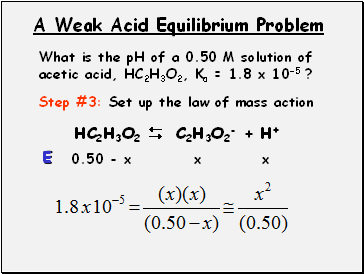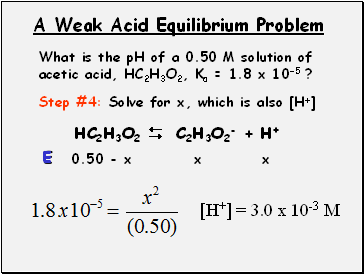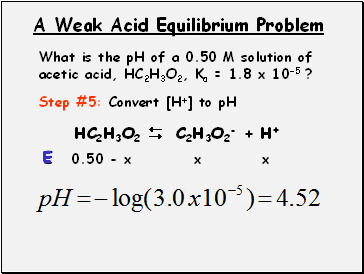# Acids, pH and EquilibriumPage 2

#### WATCH ALL SLIDES

A Weak Acid Equilibrium Problem

What is the pH of a 0.50 M solution of acetic acid, HC2H3O2, Ka = 1.8 x 10-5 ?

Step #2: ICE it!

HC2H3O2  C2H3O2- + H+

0.50

0

0

- x

+x

+x

0.50 - x

x

x

Slide 14A Weak Acid Equilibrium Problem

What is the pH of a 0.50 M solution of acetic acid, HC2H3O2, Ka = 1.8 x 10-5 ?

Step #3: Set up the law of mass action

HC2H3O2  C2H3O2- + H+

0.50 - x

x

x

E

Slide 15A Weak Acid Equilibrium Problem

What is the pH of a 0.50 M solution of acetic acid, HC2H3O2, Ka = 1.8 x 10-5 ?

Step #4: Solve for x, which is also [H+]

HC2H3O2  C2H3O2- + H+

0.50 - x

x

x

E

[H+] = 3.0 x 10-3 M

Slide 16A Weak Acid Equilibrium Problem

What is the pH of a 0.50 M solution of acetic acid, HC2H3O2, Ka = 1.8 x 10-5 ?

Step #5: Convert [H+] to pH

HC2H3O2  C2H3O2- + H+

0.50 - x

x

x

E

Go to page:
1  2